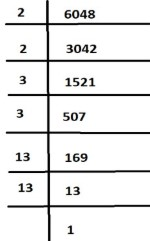QUESTION

# Find the square root of 6084 by division method.$\therefore 2 \times 2 \times 3 \times 3 \times 13 \times 13 = 6048$
$\therefore {2^2}{.3^2}{.13^2} = 6048$, we have to calculate the square root
$\therefore \sqrt {{2^2}{{.3}^2}{{.13}^2}} = \sqrt {6048}$
$\Rightarrow 2 \times 3 \times 13 = \sqrt {6048} = 78$